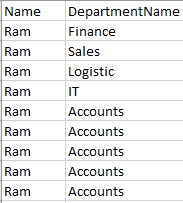# Data Table question

Hi Guys,
I have a datatable contains 2 columns(Employee Name(some are same) & Department Name).
I want to find how many employees having same name as Ram Mentioned in below snipHello @Nitin2606,

1. dt.rows.count - will return integer no,
2. dt = dt.select(“Emp name= ‘Ram’”).CopyToDatatable
3. dt.rows.count - this will give us a ram’s count

Cheers,
Pankaj

1 Like

Thanks @Pankaj.Patil Pankaj for the reply.

I am new to this so i have first given the path using excel application scope and then read the whole range using read range activity.
please let me further which activity i can use to get the result.

So your source is a excel i suppose. Read the excel and the data will get stored in a datatable.

1. Add a For Each Row activity

2. Create a variable “VName” as String data type. (Name can be anything)
Within the loop

• Use Assign activity to capture the value of the current row. Assign the value to the variable you created. To capture the value, use below
`Row("Name").ToString`

• Use another assign activity within the loop to capture the count of that value. This will give the count of each name. To get the count use below

`YourDataTable.Select("Name = '" + VName + "'").Count` - Vname is the variable we created above to hold the name of each row within the loop

1 Like

First Read the Csv File and in the ouput create the one datatable variable use this variable in for each row Select the column using get row item or using the column index. then next use the if condition Use the Condition as “YourGetrowItemValue = Ram” Then in the then section you can count the rows using Yourdatatablevariable.Rows.count(row). this you can calculate how many Ram are there.

hi @Nitin2606
here’s my workflow,
take a look.

countRam.zip (10.8 KB)

Thanks Guys

1 Like

thanks

thanks @Lahiru.Fernando

1 Like